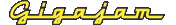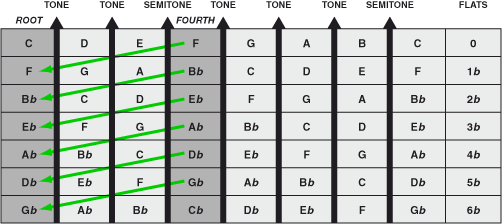EMT Lesson Four  How to understand keys and key signatures

# Cycle of fourths — the more common way to calculate flat key signatures

It should also be noted that, when calculating the notes in the flat (b) key, a more commonly used method is to go up a fourth (five semitones above the previous note) to calculate the key signature – as an alternative to going down a fifth. This is known as the ‘cycle of fourths’.

Figure 19. The cycle of fourths (keys with flats in the key signature)   (Enlarge)Next: Circle of fifths